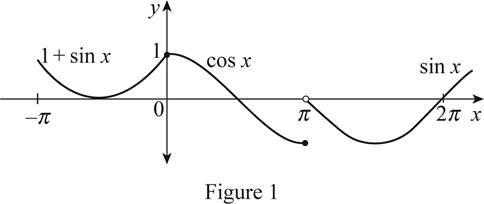# The graph of f ( x ) and determine the value of a from the graph for which lim x → a f ( x ) exists.### Single Variable Calculus: Concepts...

4th Edition
James Stewart
Publisher: Cengage Learning
ISBN: 9781337687805### Single Variable Calculus: Concepts...

4th Edition
James Stewart
Publisher: Cengage Learning
ISBN: 9781337687805

#### Solutions

Chapter 2.2, Problem 8E
To determine

## To sketch: The graph of f(x) and determine the value of a from the graph for which limx→af(x) exists.

Expert Solution

### Explanation of Solution

Given:

The function f(x)={1+sinxif x<0cosxif 0xπsinxif x>π.

Graph:

Draw the graph of f(x) as follows.

Draw the graph of 1 + sin x curve to the left of vertical axis since the interval is restricted that x < 0. Then draw the cosine curve between the interval 0xπ and then draw the sine curve for the interval x>π.

The graph of f(x) is shown below in Figure 1.From Figure 1, it is observed that the graph of f(x)=1+sinx lies to the left of the vertical axis, the graph of f(x)=cosx lies to the left of x=π and the graph of f(x)=sinx the lies to the right of x=π.

Also notice that, limx0f(x)=limx0+f(x)=1 and limxπf(x)=1,limxπ+f(x)=0.

Hence, limxπf(x) does not exist.

Therefore, it can be concluded that limxaf(x) exists every values of a excluding a=π.

### Have a homework question?

Subscribe to bartleby learn! Ask subject matter experts 30 homework questions each month. Plus, you’ll have access to millions of step-by-step textbook answers!